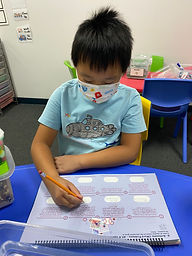## Ms. Briana

### Target 1​

###### Lesson Type:

Continuation

Number Operation

:

Computation

Solve multi-step word problems within 100 (addition, subtraction, multiplication, and division).

###### 1:

Identify the correct arithmetic processes based on the information presented in word problems.

###### 2:

Find the key words in word problems that indicate the correct arithmetic process to use.

###### 3:

Find and use the needed information in a word problem in order to solve.

###### 4:

Write an equation from a word problem with a variable included in the equation. Then solve for the variable.

4th

###### Vocabulary:

Variable, CUBES

Activities:

Students reviewed CUBES method and practiced problems together on the board.

Students praceticed CUBES method solving word problems in their work books.### Home Exploration

###### Guiding Questions:## Absent Students:

### Target 2

:

###### 1:

Identify that weight can be measured with scales, balances, etc.

###### 2:

Understand equivalent measurements.

###### 3:

Identify that capacity can be measured with measuring cups, cylinders, etc.

6th

###### Vocabulary:

Balances, Scales, Cylinders, Capasity, Equivalent

Activities:

Studentrs explored measureing cups with pom poms, water and beans/rice and etc.

Students used a balance scale to compare the weight of different items and create equivalent weights.

Students used different recepticals to measure and compare capasity.

Students used fraction measuring cups full of water with the balance scale to see the fractions relate to each other. (Ex: one side of the scale has 1 cup of water and on the other side we added 1/2+1/4+14 cup. The students then saw both sides are balanced)### Home Exploration

###### Guiding Questions:### Target 3

:

###### Vocabulary:

Activities:### Home Exploration